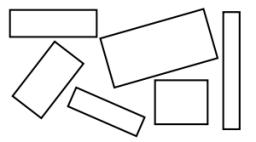# Rectangles 6279

Reduce the dimensions of the rectangle with dimensions of 21 cm and 1.4 dm in a ratio of 4:7. In what proportion will the areas of both rectangles be?

r =  49:16 cm

### Step-by-step explanation:Did you find an error or inaccuracy? Feel free to write us. Thank you!

Showing 1 comment:
Math student
If a = 12 cm, b = 27 cm, c = 16 cm, and d = 20 cm, what is the area of the poster? what is the answer

Tips for related online calculators
Need help calculating sum, simplifying, or multiplying fractions? Try our fraction calculator.
Check out our ratio calculator.
Do you want to convert length units?

#### Grade of the word problem:

We encourage you to watch this tutorial video on this math problem: